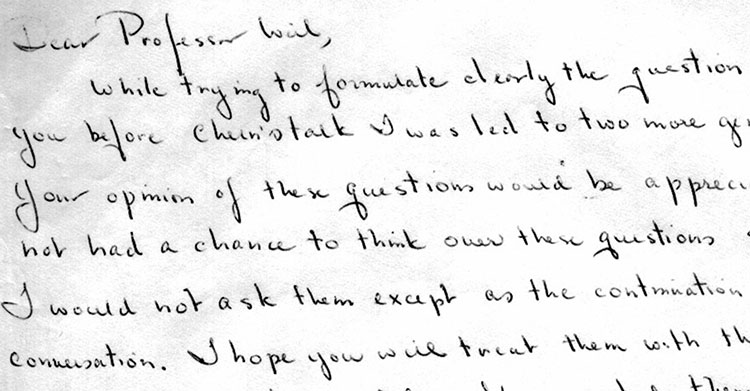# Tag: Langlands

The Bulletin of the AMS just made this paper by Julia Mueller available online: “On the genesis of Robert P. Langlands’ conjectures and his letter to Andre Weil” (hat tip +ChandanDalawat and +DavidRoberts on Google+).

It recounts the story of the early years of Langlands and the first years of his mathematical career (1960-1966)leading up to his letter to Andre Weil in which he outlines his conjectures, which would become known as the Langlands program.Langlands letter to Weil is available from the IAS.

The Langlands program is a vast net of conjectures. For example, it conjectures that there is a correspondence between

– $n$-dimensional representations of the absolute Galois group $Gal(\overline{\mathbb{Q}}/\mathbb{Q})$, and

– specific data coming from an adelic quotient-space $GL_n(\mathbb{A}_{\mathbb{Q}})/GL_n(\mathbb{Q})$.

For $n=1$ this is essentially class field theory with the correspondence given by Artin’s reciprocity law.

Here we have on the one hand the characters of the abelianised absolute Galois group

$Gal(\overline{\mathbb{Q}}/\mathbb{Q})^{ab} \simeq Gal(\mathbb{Q}(\pmb{\mu}_{\infty})/\mathbb{Q}) \simeq \widehat{\mathbb{Z}}^{\ast}$

and on the other hand the connected components of the idele class space

$GL_1(\mathbb{A}_{\mathbb{Q}})/GL_1(\mathbb{Q}) = \mathbb{A}_{\mathbb{Q}}^{\ast} / \mathbb{Q}^{\ast} = \mathbb{R}_+^{\ast} \times \widehat{\mathbb{Z}}^{\ast}$

For $n=2$ it involves the study of Galois representations coming from elliptic curves. A gentle introduction to the general case is Mark Kisin’s paper What is … a Galois representation?.

One way to look at some of the quantum statistical systems studied via non-commutative geometry is that they try to understand the “bad” boundary of the Langlands space $GL_n(\mathbb{A}_{\mathbb{Q}})/GL_n(\mathbb{Q})$.

Here, the Bost-Connes system corresponds to the $n=1$ case, the Connes-Marcolli system to the $n=2$ case.

If $\mathbb{A}’_{\mathbb{Q}}$ is the subset of all adeles having almost all of its terms in $\widehat{\mathbb{Z}}_p^{\ast}$, then there is a well-defined map

$\pi~:~\mathbb{A}’_{\mathbb{Q}}/\mathbb{Q}^{\ast} \rightarrow \mathbb{R}_+ \qquad (x_{\infty},x_2,x_2,\dots) \mapsto | x_{\infty} | \prod_p | x_p |_p$

The inverse image of $\pi$ over $\mathbb{R}_+^{\ast}$ are exactly the idele classes $\mathbb{A}_{\mathbb{Q}}^{\ast}/\mathbb{Q}^{\ast}$, so we can view them as the nice locus of the horrible complicated quotient of adele-classes $\mathbb{A}_{\mathbb{Q}}/\mathbb{Q}^*$. And we can view the adele-classes as a ‘closure’ of the idele classes.

But, the fiber $\pi^{-1}(0)$ has horrible topological properties because $\mathbb{Q}^*$ acts ergodically on it due to the fact that $log(p)/log(q)$ is irrational for distinct primes $p$ and $q$.

This is why it is better to view the adele-classes not as an ordinary space (one with bad topological properties), but rather as a ‘non-commutative’ space because it is controlled by a non-commutative algebra, the Bost-Connes algebra.

For $n=2$ there’s a similar story with a ‘bad’ quotient $M_2(\mathbb{A}_{\mathbb{Q}})/GL_2(\mathbb{Q})$, being the closure of an ‘open’ nice piece which is the Langlands quotient space $GL_2(\mathbb{A}_{\mathbb{Q}})/GL_2(\mathbb{Q})$.

A few MathOverflow threads I bookmarked in 2010 for various reasons.